## Example Questions

### Example Question #3 : How To Multiply Even Numbers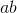is even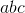is even

Therefore, which of the following must be true about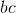?

It must be even.

It must be odd.

It could be either even or odd.

It could be either even or odd.

Explanation:

Recall that when you multiply by an even number, you get an even product.

Therefore, we know the following from the first statement:is even oris even or bothandare even.

For the second, we know this:

Sinceis even, therefore,can be either even or odd. (Regardless of what it is, we can get an even value for.)

Based on all this data, we can tell nothing necessarily about. Ifis even, thenis even, even ifis odd. However, ifis odd whileis even, thenwill be even.

### Example Question #4 : How To Multiply Even Numbers

In a group of philosophers,are followers of Durandus. Twice that number are followers of Ockham. Four times the number of followers of Ockham are followers of Aquinas. One sixth of the number of followers of Aquinas are followers of Scotus. How many total philosophers are in the group?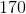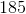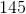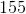Explanation:

In a group of philosophers,are followers of Durandus. Twice that number are followers of Ockham. Four times the number of followers of Ockham are followers of Aquinas. One sixth of the number of followers of Aquinas are followers of Scotus. How many total philosophers are in the group?

To start, let's calculate the total philosophers:

Ockham:* <Number following Durandus>, or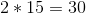Aquinas:* <Number following Ockham>, or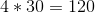Scotus:divided by, orTherefore, the total number is: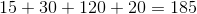### Example Question #22 : Even / Odd Numbers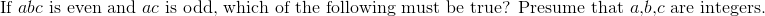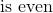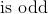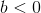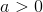Explanation:

We know that when you multiply any integer by an even number, the result is even. Thus, if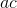is odd but when you multiply this bythe numberis even, we know thatmust be even. You cannot say anything about the sign value of any of the numbers. Likewise, it is impossible for eitherorto be even.

### Example Question #23 : Even / Odd Numbers

The product of three consecutive nonzero integers is taken. Which statement must be true?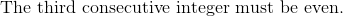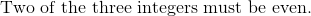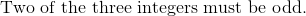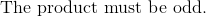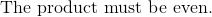Explanation:

Three consecutive integers will include at least one, and possibly two, even integers. Since the addition of even a single even integer to a chain of integer products will make the final product even, we know the product must be even.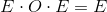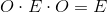### Example Question #1 : How To Multiply Even Numbers

Which of the following integers has an even integer value for all positive integers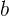and?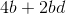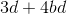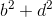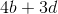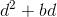There are certain patterns that can be used to predict whether the product or sum of numbers will be odd or even. The sum of two odd numbers is always even, as is the sum of two even numbers. The sum of an odd number and an even number is always odd. In multiplication the product of two odd numbers is always odd. While the product of even numbers, as well as the product of odd numbers multiplied by even numbers is always even. So for this problem we need to find scenarios where the only possibile answers are even.can only result in even numbers no matter what positive integers are used forand, because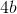must can only result in even products; the same can be said for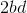. The rules provide that the sum of two even numbers is even, sois the answer.